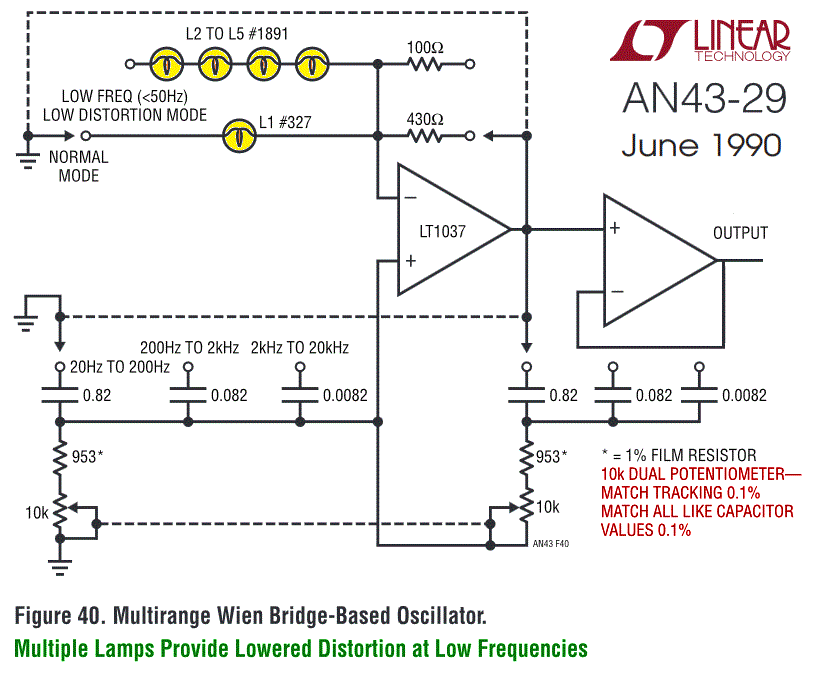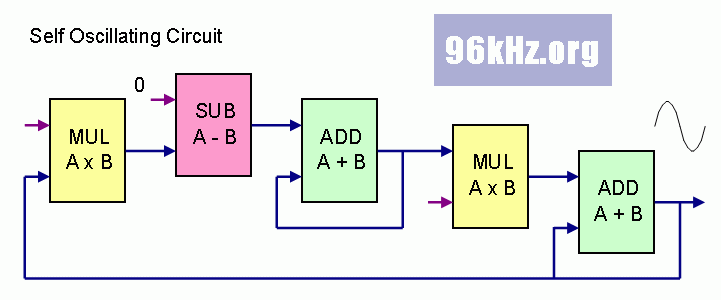96kHz.org Advanced Audio Recording A Virtual Analog Oscillator in C This page shows an example of replacing an analog circuit with digital equations.   classical analog sine circuit The following image shows an interesting analog circuit to produce accurate sine waves with, presented in the LINEAR design journal 10 years ago. It has a remarkable distortion of less than 0,025%. Funnily it uses lamps to smoothly control the current in the path and linearize its behavior especially for low frequencies. Never tried to build it but WIEN bridge circuits generally come with high quality sine waves indeed.a self oscillating circuit Such an oscillator equivalent can easily be built using two accumulators with a feedback and scaled factors in the path, realized with simple mathematical functions (dy/dt and dy/dt2):The adders accumulate the incoming scaled values and thus act as integrators. While the first accumulated value represents a kind of "velocity", the second is the "position" = "stress" in the system and directly determines the inverted acceleration. The resulting equations for this case are a classical differential equation system and result in a sine wave - the familiar solution for oscillating mechanical and electrical systems. To use this directly in the C language, it is convenient to use the REAL type in the calculations for the variables. In this way, an accuracy of better than 0.002% can be achieved for audio signals with processor frequencies of 10MHz and more, as used in current AVR.   virtual oscillating circuit in integer For INTEGER calculation see this page here: One has to use intelligent rounding and noising techniques to obtain a sufficient accuracy and avoid issues caused by vector truncation: Virtual Analog Oscillator © 2002 J.S.